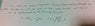# Maxwell boltzmann distribution

• MissP.25_5

#### MissP.25_5

The question is in the image file. I am stuck, I don't know how to start. Can someone guide me please?

#### Attachments

•IMG_5154.jpg
13.2 KB · Views: 393
The question is in the image file. I am stuck, I don't know how to start. Can someone guide me please?

As far as I can tell, this is a geometry problem that can be done by appropriately integrating over solid angles. The molecules are traveling with the same average velocities in all directions. Anyway, integrating the over all solid angles for a hemisphere to calculate the flux normal to a plane oriented perpendicular to the x-axis is the way I would approach this problem. But, there may be simpler ways.

Chet

I checked on the internet, and found the correct way of doing this. Let N represent the number of molecules per unit volume and let p(vx)dvx represent the fraction of the molecules with x velocities between vx and vx+dvx. The number of molecules with velocities in this velocity interval per unit area perpendicular to the x-axis per unit time is Nvxp(vx)dvx. The total number of molecules per unit area per unit time over all velocity intervals is the integral of this from vx=0 to vx-->∞. So the key to all this is to know p(vx). Do you know this x velocity distribution function for the MB velocity distribution?